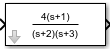# droots

Display transfer function on masked subsystem icon

## Syntax

```droots(`zero`, `pole`, `gain`)
droots(`zero`, `pole`, `gain`,'z')
droots(`zero`, `pole`, `gain`,'z-')
```

## Description

`droots(zero, pole, gain)` displays the transfer function whose zero is `zero`, pole is `pole`, and gain is `gain`.

`droots(zero, pole, gain,'z') `and `droots(zero, pole, gain,'z-')` expresses the transfer function in terms of z or 1/z.

When Simulink® draws the block icon, the initialization commands execute and the resulting equation appears on the block icon, as in the following examples:

• To display a zero-pole gain transfer function, enter

```droots(z, p, k) ```

For example, the preceding command creates this icon for these values:

```z = []; p = [-1 -1]; k = 1; ```If the parameters are not defined or have no values when you create the icon, Simulink software displays three question marks (`? ? ?`) in the icon. When you define parameter values in the Mask Settings dialog box, Simulink software evaluates the transfer function and displays the resulting equation in the icon.

## Version History

Introduced in R2007a